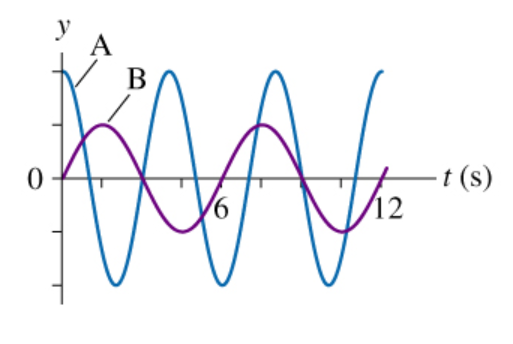# Problem: The two graphs in (Figure 1) are for two different vertical mass/spring systems.Part AWhat is the frequency of system A?Express your answer using two significant figures.Part BWhat is the first time at which the mass of system A has maximum speed while traveling in the upward direction?Express your answer using two significant figures.Part CWhat is the period of system B?Express your answer using two significant figures.Part DWhat is the first time at which the mechanical energy of system B is all potential?Express your answer using two significant figures.Part EIf both systems have the same mass, what is the ratio kA/kB of their spring constants?Express your answer using two significant figures.

###### FREE Expert Solution

Frequency:

$\overline{){\mathbf{f}}{\mathbf{=}}\frac{\mathbf{1}}{\mathbf{T}}}$

The time period of the mass of a spring system is expressed as:

$\overline{){\mathbf{T}}{\mathbf{=}}{\mathbf{2}}{\mathbf{\pi }}\sqrt{\frac{\mathbf{m}}{\mathbf{k}}}}$

Part A

TA = 4 s

fA = 1/4 = 0.25 Hz

The frequency of system A is 0.25 Hz.

86% (30 ratings)###### Problem Details

The two graphs in (Figure 1) are for two different vertical mass/spring systems.Part A

What is the frequency of system A?

Part B

What is the first time at which the mass of system A has maximum speed while traveling in the upward direction?

Part C

What is the period of system B?

Part D

What is the first time at which the mechanical energy of system B is all potential?

Part E

If both systems have the same mass, what is the ratio kA/kB of their spring constants?